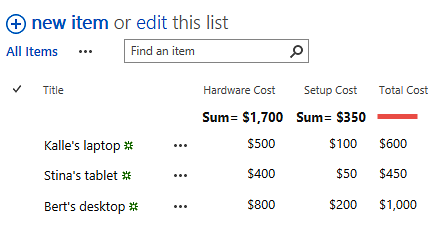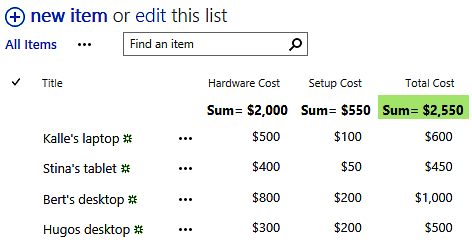Google

## 05 March 2019

### SharePoint Totals in Both Rows and ColumnsWould you like to see the total of sums or numbers both vertically and horizontally in a SharePoint list? That is possible if you let a flow or workflow calculate the row total and let the SharePoint built-in Totals view show totals for the columns. I have described how to do it in two Tips articles on the kalmstrom.com website.

The built-in Totals view
The SharePoint ‘Totals’ feature can be used to summarize the values in a column. It can also calculate other values for number columns, like average, maximum and minimum. The result of the calculation is shown on top of the column that is calculated.

The Totals value is however only displayed in the standard mode of the classic interface, not in the Quick Edit mode or in the modern interface. I hope Microsoft will soon add Totals to the modern interface too, because it is a really useful feature.

No totals for calculated columns
If you want to use totals, you cannot use a calculated column, because it is not possible to use the Totals view on a column with calculated values. This means that you cannot get the total for the 'Total Cost' column in the image below, as that is a calculated column that gets its values from calculations of the values in the two other columns.Instead, you can use a currency or number column and let a flow or workflow do the calculation. Then it is possible to use the Totals view, and you will have totals both vertically and horizontally.Microsoft FlowIf you want to let a flow do the calculation, use an expression that calculates the sum of column values for a list item. You can create such an expression by entering the function ‘add’ and then the dynamic content for the columns that should be calculated. Separate the columns with a comma.

SharePoint workflowYou can of course also let a workflow calculate column values. First, create a local variable that gets its value from a ‘Do Calculation’ action. Then use this variable in a 'Set Field in Current Item' action to update the column with the totals value for the created or changed list item.

Books
I have created the articles and the demos above for my books on SharePoint automation. SharePoint Flows from Scratch has already been published on Amazon, and I hope to release SharePoint Workflows from Scratch this month.

By Peter Kalmström
CEO and Systems Designer
kalmstrom.com Business Solutions